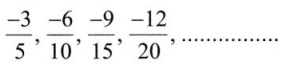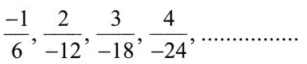# Grade 8 Rational Numbers Worksheets

## Grade 8 Maths Rational Number Multiple Choice Questions (MCQs)

1. Associative property is not followed in ……………… .
(a) whole numbers
(b) integers
(c) natural numbers
(d) none of these

2. …………………… is the identity for the addition of rational numbers.
(a) 1
(b) 0
(c) -1
(d)$\frac{1}{2}$

3. ………………… is the multiplicative identity for rational numbers.
(a) 1
(b) 0
(c) -1
(d)$\frac{1}{2}$

4. The additive inverse of$\frac{7}{5}$ is:
(a) 1
(b) 0
(c) –$\frac{7}{5}$
(d)$\frac{7}{5}$

5. What number should be added to$\frac{7}{12}$ to get$\frac{4}{15}$?
(a) –$\frac{19}{60}$
(b) –$\frac{11}{30}$
(c)$\frac{51}{60}$
(d)$\frac{1}{20}$

6. What number should be subtracted from –$\frac{3}{5}$ to get -2?
(a) –$\frac{7}{5}$
(b) –$\frac{13}{5}$
(c)$\frac{13}{5}$
(d)$\frac{7}{5}$

7. Which of the rational numbersis the smallest?
(a)$\frac{-5}{16}$
(b)$\frac{-13}{24}$
(c)$\frac{3}{-4}$
(d)$\frac{7}{-12}$

8. Rational number$\frac{3}{40}$ is equal to:
(a) 0.75
(b) 0.12
(c) 0.012
(d) 0.075

9. A rational number between$\frac{3}{5}$ and$\frac{4}{5}$ is
(a)$\frac{7}{5}$
(b)$\frac{7}{10}$
(c)$\frac{3}{10}$
(d)$\frac{4}{10}$

10. Find x such that$\frac{3}{5}=\frac{x}{-25}$
(a) -5
(b) -15
(c) -15
(d) none of these

11. Find x such that$\frac{-48}{x}$ = 2
(a) 24
(b) -12
(c) -24
(d) none of these

12. Find x such that$\frac{-3}{8}$ and$\frac{x}{-24}$ are equivalent rational numbers.
(a) 3
(b) 9
(c) 8
(d) none of these

13. Find the value of$\frac{3}{-5} \times \frac{-5}{-3}$
(a) -1
(b) 0
(c) 1
(d) none of these

14. The equivalent rational number of$\frac{-6}{5}$ is
(a)$\frac{-3}{5}$
(b)$\frac{12}{10}$
(c)$\frac{-12}{10}$
(d) none of these

15. Fill in the boxes with the correct symbol:$\frac{5}{-11} \square \frac{-5}{11}$
(a) >
(b) <
(c) =
(d) none of these

16. Fill in the boxes with the correct symbol:$\frac{1}{-3} \square \frac{-1}{4}$
(a) >
(b) <
(c) =
(d) none of these

17. Rewrite the rational number$\frac{-18}{48}$ in the simplest form.
(a)$\frac{-9}{24}$
(b)$\frac{-3}{8}$
(c)$\frac{3}{8}$
(d) none of these

18. Rewrite the rational number$\frac{44}{-72}$ in the simplest form
(a)$\frac{22}{-36}$
(b)$\frac{11}{-18}$
(c)$\frac{11}{18}$
(d) none of these

19. Write the next rational number in the pattern:(a)$\frac{12}{25}$
(b)$\frac{15}{25}$
(c)$\frac{-15}{25}$
(d) none of these

20. Write the next rational number in the pattern:(a)$\frac{-5}{-30}$
(b)$\frac{5}{-30}$
(c)$\frac{4}{-30}$
(d) none of these

### Class 8 Maths Rational Number Fill In The Blanks

1. Zero has ………………… reciprocal.
2. The numbers ……………….. and ……………….. are their own reciprocals.
3. The reciprocal of -5 is …………………… .
4. Reciprocal of$\frac{1}{x}$, where x ≠ 0 is ………………. .
5. The product of two rational number is always a ……………. .
6. The reciprocal of a positive rational number is ……………… .
7. The additive inverse of$\frac{-a}{b}$ is ………………… .
8. Reciprocal of$\frac{-3}{4}$ is ……………… .
9. The reciprocal of a negative rational number is ……………… rational number.
10. The rational number ……………… is equal to its negative.

### Class 8 Maths Rational Number Very Short Answer Type Questions

1. What is the additive inverse of$\frac{-7}{11}$?
2. What is the multiplicative inverse of$2 \frac{3}{11} \times \frac{1}{3}$?
3. Which number lies between$\frac{1}{5}$ and$\frac{1}{4}$?
4. Find the product of$\frac{3}{8}$ and the reciprocal of$\frac{-5}{17}$.
5. A tailor needs$\left(\frac{9}{10}\right)^{t h}$ of a metre of cloth to stitch a blouse. It he wants to stitch 100 blouses how much cloth will be needed?
6. The sum of the additive inverse and multiplicative inverse of 3 is ………………. .

### Class 8 Maths Rational Number Short Answer Type Questions

1. The number which is subtracted from$\frac{29}{13}$ to get$\frac{-5}{7}$ is ………………. .
2. The value of x for which the two rational numbers$\frac{4}{9}$,$\frac{x}{54}$ are equivalent.
3.$\frac{5}{6}$ of all the money in Salma’s bank account is ₹ 55,000. How much money does Salma have in her bank account?
4. Arrange the number$\frac{1}{4}$,$\frac{13}{16}$ and$\frac{5}{8}$ in the ascending order.

### Class 8 Maths Rational Number Long Answer Type Questions

1. Simplify:2. The cost of 3$\frac{1}{2}$ kg of wheat is ₹ 63. How much will 1$\frac{1}{4}$ kg of wheat cost?
3. Season tickets usually cost ₹ 120$\frac{4}{5}$ per person. For students, they are priced at$\frac{1}{4}$ of the normal cost. How much will 6 tickets will cost for students?